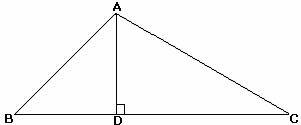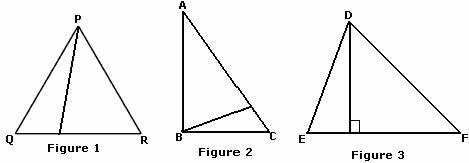## Definition Of Altitude Of A Triangle

Altitude of a Triangle is the perpendicular distance from any of its vertices to the opposite side. (This opposite side of the vertex is called the base of the altitude.)

### More about Altitude of a Triangle

• As a triangle has 3 vertices, it has 3 altitudes.
• Altitudes can be used to find the area of the triangle.

### Examples of Altitude of a TriangleIn the triangle ABC, AD is the perpendicular distance drawn from vertex A to the side BC. So, AD is the altitude of the triangle.

### Solved Example on Altitude of a Triangle

#### Ques: Which of the figures shows an altitude of the triangle drawn?A. Figure 1
B. Figure 2
C. Figure 3
D. none of these